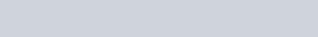Wheatstone Bridge Circuit Diagram, Applications, Formula – Class 12, JEE & NEET
The Wheatstone Bridge circuit is nothing more than two simple series-parallel arrangements of resistances connected between a voltage supply terminal and ground producing zero voltage difference between the two parallel branches when balanced. Here we will study about, Wheatstone Bridge, the Conditions of balanced and Unbalanced Wheatstone bridge circuit Diagram, and its applications.

It is an arrangement of four resistances devised by Charles Wheatstone which is used to measure an unknown resistance.

### Principle of Wheatstone Bridge

The Wheatstone bridge principle states that if four resistances P, Q, R, and S are arranged to form a bridge with a cell and key between A and C, and a galvanometer between B and D then the bridge is said to be balanced when the galvanometer shows a zero deflection.

In balanced condition$\mathrm{I}_{9}=0 \quad$ so $\mathrm{V}_{\mathrm{B}}=\mathrm{V}_{\mathrm{D}}$ or $\frac{P}{Q}=\frac{R}{s}$. This is called condition of balance.### Balanced Wheatstone Bridge

The condition of balance depends on resistance P, Q, R, and S. This is independent of the emf of the battery.

In-state of balance, the cell and galvanometer can be interchanged. While performing an experiment at start press the cell key $K_{c}$ first and then the galvanometer key $\mathrm{K}_{9}$ and at end remove $\mathrm{K}_{9}$ first and then $\mathrm{K}_{\mathrm{c}}$ to avoid induced effects.

P and Q are called ratio arms, R is the known resistance arm and S is the unknown arm. BD and AC are called conjugate arms.

The resistance of a balanced Wheatstone bridge between A and C is $\frac{(P+Q)(R+S)}{P+Q+R+S}$.

The sensitivity of the bridge depends upon the value of resistances. The sensitivity is maximum when P, Q, R, and S are of the same order.

#### Unbalanced Wheatstone bridgeIf $\frac{P}{Q}<\frac{R}{s}$ then $V_{B}>V_{D}$ so current flows from $B$ to $D .$

If $\frac{P}{Q}>\frac{R}{s}$ then $V_{B}<V_{D}$ so current flows from $D$ to $B$

### Applications of Wheatstone Bridge

The Wheatstone Bridge has many uses in electronic circuits other than comparing an unknown resistance with a known resistance. When used with Operational Amplifiers, the Wheatstone bridge circuit can be used to measure and amplify small changes in resistance, RX due.

Wheatstone bridge is not suitable for measurement of very small and very high resistances. Very low resistances are measured by Kelvin’s double bridge while very high resistances by leakage method.

For Complete Study Material and Video Tutorials for JEE & NEET Exam Preparation Download eSaral APPAlso Read: